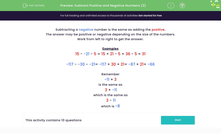# Subtract Three Positive and Negative Numbers

In this worksheet, students will subtract three positive and negative numbers.Key stage:  KS 3

Curriculum topic:   Number

Curriculum subtopic:   Use Four Operations for All Numbers

Difficulty level:#### Worksheet Overview

Subtracting a negative number is the same as adding the positive.

The answer may be positive or negative depending on the size of the numbers.

Work from left to right to get the answer.

Examples

15 - -21 - 5 = 15 + 21 - 5 = 36 - 5 = 31

-117 - -30 - -21= -117 + 30 + 21= -87 + 21= -66

Remember

-11 + 3

is the same as

3 + -11

which is the same as

3 - 11

which is -8

These may look complicated, but the key is to remember the rules for negative numbers - two negatives next to each other make a positive!!

Let's try some questions now.

### What is EdPlace?

We're your National Curriculum aligned online education content provider helping each child succeed in English, maths and science from year 1 to GCSE. With an EdPlace account you’ll be able to track and measure progress, helping each child achieve their best. We build confidence and attainment by personalising each child’s learning at a level that suits them.

Get started